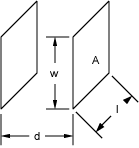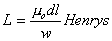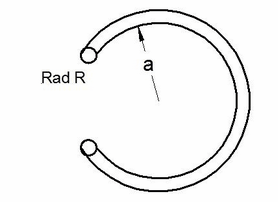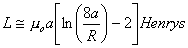NESS ENGINEERING
TECHNICAL DATA
INDUCTANCE

The formulas on this page allow one to calculate the inductance for certain given geometries.  More complex problems typically require one to model the geometry and solve using Finite Element Analysis.  The viewer should note that the permeability has been set to that of free space for these formulas since that is typically the most common scenario in calculating the inductance of a transmission line, cable, etc.

Other inductance formulas can also be found in the following references:

Inductance Calculations Working Formulas and Tables, Frederick W. Grover, Dover Publications, Inc., New York, New York, 1946.

Radio Engineers Handbook, Frederick E. Terman, McGraw-Hill Book Company, New York, New York, 1943.

"Formulas for the Skin Effect", Harold A. Wheeler, Proceedings of the I.R.E., September, 1942, pp. 412-424.

2 Parallel Plane Platesm0 is the permeability of free space (H/m)  d is the distance between the plates (m)  l is the length of the electrodes (m)  w is the width of the electrodes (m)

2 Coaxial Cylindersm0 is the permeability of free space (H/m)  a is the radius of the inner cylinder  b is the radius of the outer cylinder  l is the length of the cylinders (m)

Wire Loopm0 is the permeability of free space (H/m)  a is the radius of the wire loop (m)  R is the radius of the wire (m)

Send consulting inquiries, comments, and suggestions to nessengr@san.rr.com

Ness Engineering, Inc.
P.O. Box 261501
San Diego, CA 92196
(858) 566-2372
(858) 240-2299 FAX

 © Richard M. Ness and Ness Engineering, Inc. Last Updated: